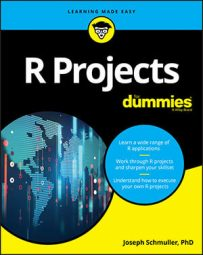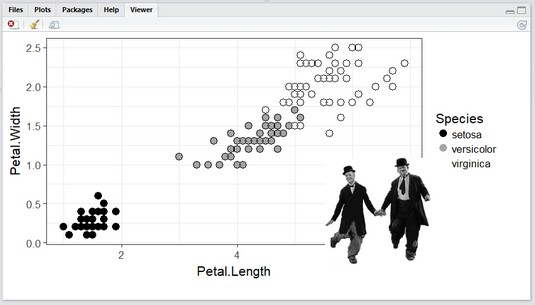##### R Projects For DummiesIf you’ve been working with images, animated images, and combined stationary images in R, it may be time to take the next step. This project walks you through the next step: Combine an image with an animated image.

This image shows the end product — the plot of the `iris` data set with comedy icons Laurel and Hardy positioned in front of the plot legend. When you open this combined image in the Viewer, you see Stan and Ollie dancing their little derbies off. (The derbies don’t actually come off in the animation, but you get the drift.)Laurel and Hardy, dancing in front of the legend in the `iris` plot.

## Getting Stan and Ollie

Check out the Laurel and Hardy GIF. Right-click the image and select Save Image As from the pop-up menu that appears. Save it as `animated-dancing-image-0243` in your `Documents` folder.

`l_and_h <- image_read("animated-dancing-image-0243.gif")`

Applying the `length()` function to `l_and_h`

`> length(l_and_h)`

` 10`

indicates that this GIF consists of ten frames.

To add a coolness factor, make the background of the GIF transparent before `image_read()` works with it. This free online image editor does the job quite nicely.

## Combining the boys with the background

If you use the image combination technique, the code looks like this:

`image_composite(image=background, composite_image=l_and_h, offset = "+510+200")`

The picture it produces looks like the image above but with one problem: The boys aren’t dancing. Why is that?

The reason is that `image_composite()` combined the `background` with just the first frame of `l_and_h`, not with all ten. It’s exactly the same as if you had run

```image_composite(image=background, composite_image=l_and_h,
offset = "+510+200")```
The `length()` function verifies this:
```> length(image_composite(image=background, composite_image=l_and_h,
offset = "+510+200"))
 1```
If all ten frames were involved, the `length()` function would have returned 10.

To get this done properly, you have to use a `magick` function called `image_apply()`.

## Explaining image_apply()

So that you fully understand how this important function works, let's describe an analogous function called `lapply()`.

If you want to apply a function (like `mean()`) to the variables of a data frame, like `iris`, one way to do that is with a `for` loop: Start with the first column and calculate its mean, go to the next column and calculate its mean, and so on until you calculate all the column means.

For technical reasons, it’s faster and more efficient to use `lapply()` to apply `mean()` to all the variables:

```> lapply(iris, mean)
\$Sepal.Length
 5.843333\$Sepal.Width
 3.057333\$Petal.Length
 3.758\$Petal.Width
 1.199333\$Species
 NA```
A warning message comes with that last one, but that’s okay.

Another way to write `lapply(iris, mean)` is `lapply(iris, function(x){mean(x)})`.

This second way comes in handy when the function becomes more complicated. If, for some reason, you want to square the value of each score in the data set and then multiply the result by three, and then calculate the mean of each column, here’s how to code it:

`lapply(iris, function(x){mean(3*(x^2))})`

In a similar way, `image_apply()` applies a function to every frame in an animated GIF. In this project, the function that gets applied to every frame is `image_composite()`:

`function(frame){image_composite(image=background, composite_image=frame, offset = "+510+200")}`

So, within `image_apply()`, that’s

```frames <- image_apply(image=l_and_h, function(frame) {
image_composite(image=background, composite_image=frame, offset = "+510+200")
})```
After you run that code, `length(frames)` verifies the ten frames:

`> length(frames)` ` 10`

## Getting back to the animation

The `image_animate()` function puts it all in motion at ten frames per second:

`animation <- image_animate(frames, fps = 10)`

To put the show on the screen, it’s

`print(animation)`

All together now:

```l_and_h <- image_read("animated-dancing-image-0243.gif")
background <- image_background(iris_plot, "white)frames <- image_apply(image=l_and_h, function(frame) {
image_composite(image=background, composite_image=frame, offset = "+510+200")
})animation <- image_animate(frames, fps = 10)
print(animation)```
And that’s the code for the image above.

One more thing. The `image_write()` function saves the animation as a handy little reusable GIF:

`image_write(animation, "LHirises.gif")`